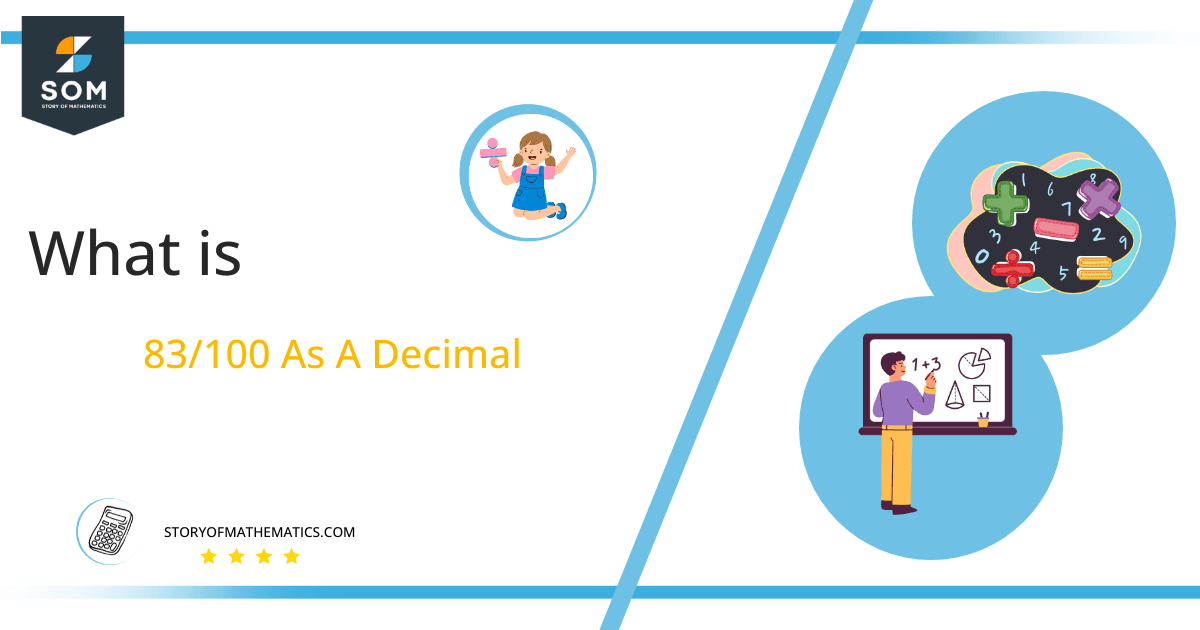# What Is 83/100 as a Decimal + Solution With Free Steps

The fraction 83/100 as a decimal is equal to 0.83.

When there is a link between two numbers that includes Division, a Fraction is employed to represent them. There are several methods for solving the fraction, but when one number is not completely divided by another we prefer long divisi0n method.

Here, we are interested more in the types of division that results in a Decimal value, as this can be expressed as a Fraction. We see fractions as a way of showing two numbers having the operation of Division between them that result in a value that lies between two Integers.Now, we introduce the method used to solve said fraction to decimal conversion, called Long Division which we will discuss in detail moving forward. So, let’s go through the Solution of fraction 83/100.

## Solution

First, we convert the fraction components i.e., the numerator and the denominator, and transform them into the division constituents i.e., the Dividend and the Divisor respectively.

This can be seen done as follows:

Dividend = 83

Divisor = 100

Now, we introduce the most important quantity in our process of division, this is the Quotient. The value represents the Solution to our division, and can be expressed as having the following relationship with the Division constituents:

Quotient = Dividend $\div$ Divisor = 83 $\div$ 100

This is when we go through the Long Division solution to our problem.Figure1

## 83/100 Long Division Method

We start solving a problem using the Long Division Method by first taking apart the division’s components and comparing them. As we have 83, and 100 we can see how 83 is Smaller than 100, and to solve this division we require that 83 be Bigger than 100.

This is done by multiplying the dividend by 10 and checking whether it is bigger than the divisor or not. If it is then we calculate the Multiple of the divisor which is closest to the dividend and subtract it from the Dividend. This produces the Remainder which we then use as the dividend later.

Now, we begin solving for our dividend 83 , which after getting multiplied by 10 becomes 830.

We take this 830 and divide it by 100, this can be seen done as follows:

830 $\div$ 100 $\approx$ 8

Where:

100 x 8 = 800

This will lead to the generation of a Remainder equal to 830 – 800 = 30, now this means we have to repeat the process by Converting the 30 into 300 and solving for that:

300 $\div$ 100 = 3

Where:

100 x 3 = 300

Finally, we have a Quotient generated after combining the three pieces of it as 0.83 = z, with a Remainder equal to 0.Images/mathematical drawings are created with GeoGebra.